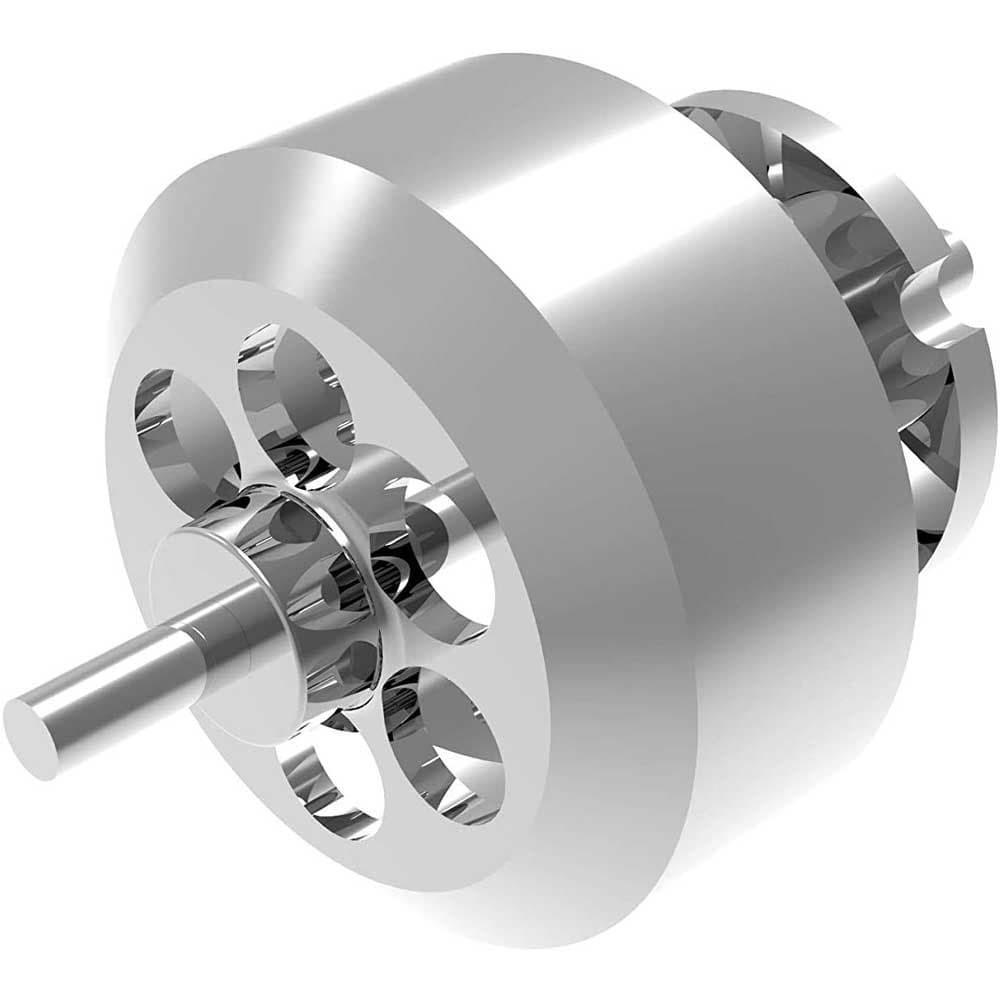Brushless motors use a standard numbering scheme to describe their physical size and Kv rating. For example: let's assume we have a 5055-3000Kv Brushless Outrunner Motor. We break the numbers out as follows:    - 

•  The first two numbers represent the diameter of the motor's housing in millimeters; in this example 50mm
•  The second two numbers represent the length of the motor housing in millimeters; in this example 55mm
•  The numbers after the dash represent the Kv rating of the motor; in this example 3000Kv. The Kv rating (not to be confused with kilo-volt) is the RPM of the motor (k) per volt (V) with no load. For example, a brushless motor with a Kv rating of 3000 powered by a 12V power source would be capable of 36,000 RPMs (multiply 3000x12). This is the max RPMs that this motor can reach under no load. A motor with a higher Kv will have more top end speed, but not as much acceleration/torque. A motor with a lower Kv will not be as fast, but will accelerate faster and have more torque.